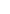LCOV - code coverage reportCurrent view: top level - py - modmath.c (source / functions) Hit Total Coverage Test: unix_coverage_v1.20.0-188-gb4de697ad.info Lines: 124 124 100.0 % Date: 2023-06-09 09:48:17 Functions: 46 46 100.0 % Branches: 56 56 100.0 %Function NameHit countmp_math_expm1 32 mp_math_frexp 32 mp_math_ldexp 32 mp_math_modf 32 mp_math_acosh 36 mp_math_atanh 40 mp_math_isfinite 40 mp_math_isinf 40 fabs_func 44 mp_math_asinh 44 mp_math_atan 44 mp_math_cosh 44 mp_math_degrees 44 mp_math_erf 44 mp_math_erfc 44 mp_math_fabs 44 mp_math_radians 44 mp_math_sin 44 mp_math_sinh 44 mp_math_tan 44 copysign_func 48 mp_math_copysign 48 mp_math_cos 48 mp_math_isnan 48 mp_math_gamma 52 mp_math_log10 52 mp_math_log2 52 mp_math_sqrt 52 mp_math_acos 60 mp_math_asin 60 mp_math_atan2 60 mp_math_fmod 60 mp_math_lgamma 60 mp_math_tanh 60 mp_math_exp 64 mp_math_ceil 80 mp_math_floor 80 mp_math_trunc 80 mp_math_pow 100 mp_math_log 102 mp_math_isclose 108 mp_math_factorial 124 math_generic_2 268 math_error 280 math_generic_1 1064 mp_math_factorial_inner 1300Generated by: LCOV version 1.15-5-g462f71d